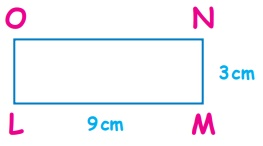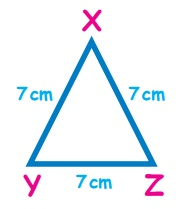Home | | Maths 4th Std | Identify the sides and find perimeter of a quadrilateral

# Identify the sides and find perimeter of a quadrilateral

Identify the sides and find perimeter of a quadrilateral

Identify the sides and find perimeter of a quadrilateral

Perimeter

The perimeter is the sum of all sides of a closed figure.

EXAMPLES

Finding the sides and perimeter of the following figures.Sides = AB, BC, CD, DA

Perimeter = AB + BC + CD + DA

= 3 + 6 + 8 + 2 = 19

Perimeter= 19cmIn a given square all the sides are equal.

Therefore, Perimeter of a square = PQ + QS + SR + RP

= 8 + 8 + 8 + 8 = 32

Perimeter of a square = 32cmIn a given rectangle opposite sides are equal.

Perimeter = LM + MN + NO + OP

= 9 + 3 + 9 + 3 = 24

Perimeter = 24cmPerimeter = XY + YZ + ZX

= 7 + 7 + 7 = 21

Perimeter = 21cm

Activity: Find the Perimeter of Table, Desk, Black board and Door in your class room.

Tags : Geometry | Term 1 Chapter 1 | 4th Maths , 4th Maths : Term 1 Unit 1 : Geometry
Study Material, Lecturing Notes, Assignment, Reference, Wiki description explanation, brief detail
4th Maths : Term 1 Unit 1 : Geometry : Identify the sides and find perimeter of a quadrilateral | Geometry | Term 1 Chapter 1 | 4th Maths